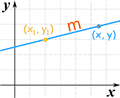# General Form of Equation of a Line

### The "General Form" of the equation of a straight line is:

Ax + By + C = 0

A or B can be zero, but not both at the same time.

The General Form is not always the most useful form, and you may prefer to use:

 The Slope-Intercept Form of the equation of a straight line: y = mx + bor The Point-Slope Form of the equation of a straight line: y − y1 = m(x − x1)y = mx + b